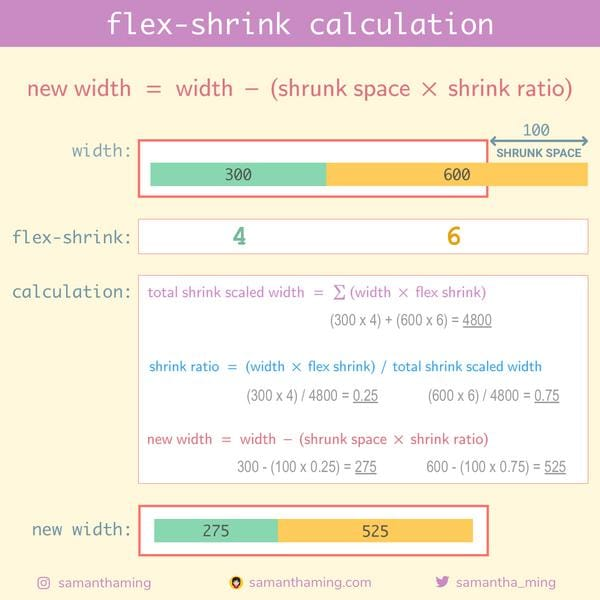# # Day 24: flex-shrink calculation

This is another optional knowledge. But if you're like me and is curious how the browser calculates flex-shrink. Join me in this rabbit hole 🐰

The math behind flex-shrink is a bit more complicated then flex-grow. You need to take into account of it's existing proportion and shrink it accordingly to the flex shrink amount. Hence, a few more calculation involved. Again, if this is throwing you off. Skip it. You don't need to know this to understand Flexbox. Luckily the browser takes care of it for you, how wonderful 😌## # Calculation

Indeed the calculation is a bit more complicated. But no worries, let's break it down we go through it step by step, you got this 💪

Here's the `HTML` and `CSS` we're working with:

HTML

``````<div class="parent">
<div class="green"></div>
<div class="yellow"></div>
</div>
``````

CSS

``````.parent {
width: 800px;
}
.green {
width: 300px;
flex-shrink: 4;
}
.yellow {
width: 600px;
flex-shrink: 6;
}
``````

### # Step 1: Breaking down the variables

This is the formula:

``````new width = width - (shrink space x shrink ratio)
``````

Let's extract the variables required in the formula to this handy table we can fill in as we go:

Variables
width need to calculate
shrink space need to calculate
shrink ratio need to calculate

### # Step 2: Fill in what we know

From the `CSS` value, we can conclude the following:

• The parent element (container) has a width of `800`
• Green child element has a width `300` and `flex-shrink` of `4`
• Yellow child element has a width `600` and `flex-shrink` of `6`

Let's update our chart with this information:

Green Yellow
flex shrink 4 6
width 300 600

### # Step 3: Calculate "shrunk space"

This is the formula:

``````shrunk space = total children widths - parent width
``````

Remember what we know:

• The parent element (container) has a width of `800`
• The child elements has a width of `300`, `600`

Great, we can use that information to calculate "total children widths":

``````total children widths = green + yellow
= 300   + 600

=> 900
``````

Now we can calculate our "shrunk space":

``````shrunk space = total children widths - parent width
= 900                   -  800

=> 100
``````

Green Yellow Total
flex shrink 4 6
width 300 600
shrunk space - - 100

### # Step 4: Calculate "shrink ratio"

This is the formula:

``````shrink ratio = (width x flex shrink) / total shrink scaled width
``````

Notice this new variable, `total shrink scaled width`. So we need to calculate that first to get our shrink ratio.

#### # Step 4-1: Calculate "total shrink scaled width"

This is the formula:

``````total shrink scaled width = Σ(width x flex shrink)
``````

"Σ" Sigma is a math symbol that means the summation of something. So we need to apply `width x flex shrink` for all the child elements.

Green

``````width x flex shrink = 300 x 4

=> 1200
``````

Yellow

``````width x flex shrink = 600 x 6

=> 3600
``````

Finally

``````total shrink scaled width = 1200 + 3600

=> 4800
``````

Let's add this information to our chart:

Green Yellow Total
flex shrink 4 6
width 300 600
shrunk space - - 100
total shrink scaled width - - 4800

#### # Step 4-2: Back to calculating "shrink ratio"

Fantastic, now that we know the "total shrink scaled width", we can return with calculating the "shrink ratio". Remember the formula:

``````shrink ratio = (width x flex shrink) / total shrink scaled width
``````

Green

``````shrink ratio = (300 x 4) / 4800

=> 0.25
``````

Yellow

``````shrink ratio = (600 x 6) / 4800

=> 0.75
``````

Let's add this information to our chart:

Green Yellow Total
flex shrink 4 6
width 300 600
shrunk space - - 100
shrink ratio 0.25 0.75

### # Final step: Calculate "new width"

Remember the formula:

``````new width = width - (shrink space x shrink ratio)
``````

Green

``````new width = 300 - (100 x 0.25)

=> 275
``````

Yellow

``````new width = 600 - (100 x 0.75)

=> 525
``````

Done! We have successfully calculated the new width 🥳

Green Yellow
width 300 600
shrunk space 4 6
shrink ratio 0.25 0.75
new width 275 525